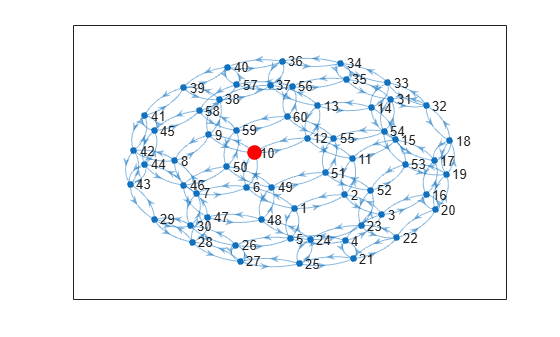# outedges

Outgoing edges from node

## Syntax

``eid = outedges(G,nodeID)``
``[eid,nid] = outedges(G,nodeID)``

## Description

example

````eid = outedges(G,nodeID)` returns the indices of all outgoing edges from node `nodeID` in graph `G`.```

example

````[eid,nid] = outedges(G,nodeID)` additionally returns the successor nodes `nid` that are connected to `nodeID` by the edges `eid`.```

## Examples

collapse all

Create an undirected multigraph with three nodes and four edges. Find the outgoing edges of node 2.

```G = graph([1 1 1 2],[2 2 3 3]); G.Edges```
```ans=4×1 table EndNodes ________ 1 2 1 2 1 3 2 3 ```
`eid = outedges(G,2)`
```eid = 3×1 1 2 4 ```

The vector `eid` contains indices to rows in the `G.Edges` table. Use the vector to index into `G.Edges`.

`G.Edges(eid,:)`
```ans=3×1 table EndNodes ________ 1 2 1 2 2 3 ```

For undirected graphs, the edges (1,2) and (2,1) are the same.

Plot a graph and highlight the outgoing edges and successors of a selected node.

Create and plot a directed graph using the `bucky` adjacency matrix. Highlight node 10 for reference.

```G = digraph(bucky); p = plot(G); highlight(p,10,'NodeColor','r','MarkerSize',10)```Determine the outgoing edges and successors of node 10. Highlight these nodes and edges.

`[eid,nid] = outedges(G,10)`
```eid = 3×1 28 29 30 ```
```nid = 3×1 6 9 12 ```
`X = G.Edges(eid,:)`
```X=3×2 table EndNodes Weight ________ ______ 10 6 1 10 9 1 10 12 1 ```
```highlight(p,nid,'NodeColor','g','MarkerSize',9) highlight(p,'Edges',eid,'EdgeColor','g')```## Input Arguments

collapse all

Input graph, specified as either a `graph` or `digraph` object. Use `graph` to create an undirected graph or `digraph` to create a directed graph.

Example: `G = graph(1,2)`

Example: `G = digraph([1 2],[2 3])`

Node identifier, specified as one of the values in this table.

ValueExample
Scalar node index`1`
Character vector node name`'A'`
String scalar node name`"A"`

Example: `outedges(G,1)`

Example: `outedges(G,'A')`

## Output Arguments

collapse all

Edge indices, returned as a column vector. You can use the edge indices to index into the edges table of the graph with `G.Edges(eid,:)`.

Node IDs of successors, returned as node indices if `nodeID` is numeric, or as node names if `nodeID` is a node name. Use `findnode(G,nid)` to convert node names into node indices. You can use node indices to index into the nodes table of the graph with `G.Nodes(nid,:)`.

The node IDs in `nid` are the same as those returned by the `successors` function. However, if there are multiple outgoing edges to the same node, this node is listed more than once in `nid`.

## Tips

• By convention, for undirected graphs, all edges incident to a node are considered to be outgoing edges. Use `inedges` to find incoming edges in a directed graph.

• For graphs with multiple edges, `outedges` and `successors` can return arrays of different lengths, since there can be multiple outgoing edges to some of the successors.

## Version History

Introduced in R2018a